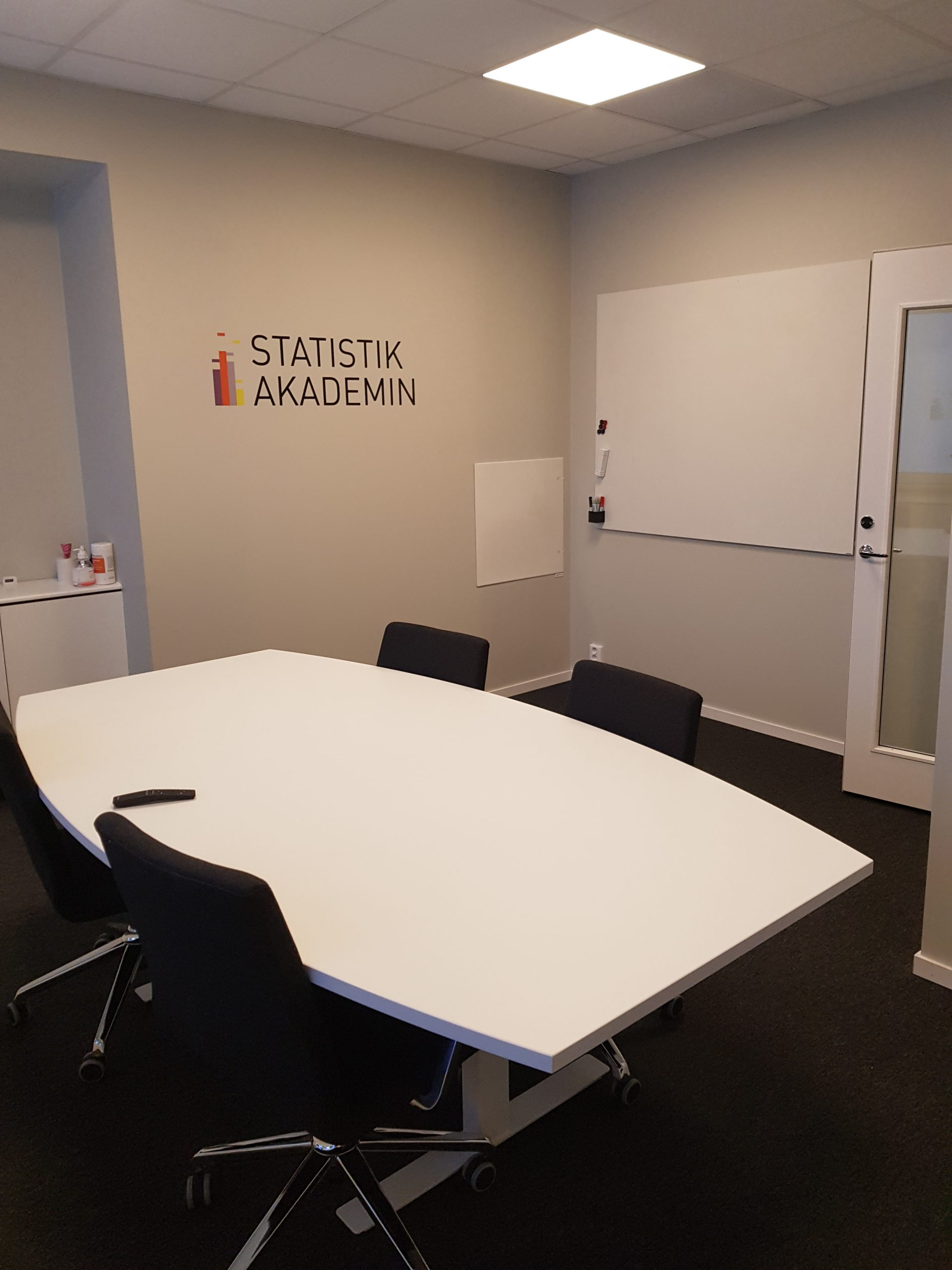## R

For you to be able to work freely in R, there are som things you need to know regardless of the intended application of the software. The basic skills required are program management, data management as well as the ability to describe data with tables and graphs.

It is also necessary to have a basic understanding of statistics in order to run accurate analyses in R. Therefore, we recommend all novice users to choose one of our course proposals.

Our proposals will help you get started as quickly as possible with both the software and the statistical models required to work independently in R. You will learn how to choose the right statistical analysis method, and how to interpret and validate the results.

## Basic

Includes
R 1
R 2

All courses are in English
A total of four days
Price: SEK 22 500

R 1

Introduction to r
Handling data
Data visualisation
Descriptive statistics

Introduction to modern statistics
Statistical concepts
Classical statistical methods
Modern statistical methods

R 2

Linear regression & ANOVA
Linear regression
ANOVA

Generalised linear models
Survival analysis
Mixed models

## Medium

Includes
R 1
R 2
R 3

All courses are in English
A total of six days
Price: SEK 31 800

R 1

Introduction to r
Handling data
Data visualisation
Descriptive statistics

Introduction to modern statistics
Statistical concepts
Classical statistical methods
Modern statistical methods

R 2

Linear regression & ANOVA
Linear regression
ANOVA

Generalised linear models
Survival analysis
Mixed models

R 3

Visualisation & data exploration
Visualisation
Dimension reduction

Cluster analysis & structural equation models
Cluster analysis
Factor analysis

## Complete

Includes
R 1
R 2
R 3

Propensity Score Matching

All courses are in English
A total of seven days
Price: SEK 36 800

R 1

Introduction to r
Handling data
Data visualisation
Descriptive statistics

Introduction to modern statistics
Statistical concepts
Classical statistical methods
Modern statistical methods

R 2

Linear regression & ANOVA
Linear regression
ANOVA

Generalised linear models
Survival analysis
Mixed models

R 3

Visualisation & data exploration
Visualisation
Dimension reduction

Cluster analysis & structural equation models
Cluster analysis
Factor analysis

Propensity Score Matching
Introduction
Identify imbalances in your data set
Matching techniques
Evaluation of the matching quality
Estimate the treatment effects
How to describe methods and results

To express your interest, please fill out the registration of interest form below.

Individual course days – Customize your reservation

There is a possibility to customize your reservation by adding individual course days. These can be added to our course proposals Basic and Medium as well as to our courses R 1–3. By adding individual course days to your reservation, you are able to tailor the training package completely according to your needs.

## Course days

Introduction to R
Introduction to modern statistics
Linear regression & ANOVA
Visualisation & data exploration
Cluster analysis & SEM
Propensity Score Matching

Introduction to r
Handling data
Data visualisation
Descriptive statistics

Introduction to modern statistics
Statistical concepts
Classical statistical methods
Modern statistical methods

Linear regression & ANOVA
Linear regression
ANOVA

Generalised linear models
Survival analysis
Mixed models

Visualisation & data exploration
Visualisation
Dimension reduction

Cluster analysis & structural equation models
Cluster analysis
Factor analysis

Propensity Score Matching
Introduction
Identify imbalances in your data set
Matching techniques
Evaluation of the matching quality
Estimate the treatment effects
How to describe methods and results